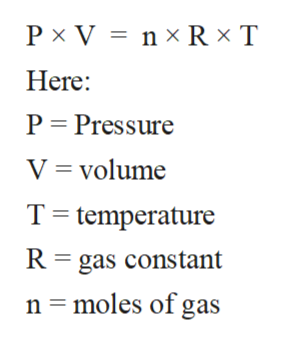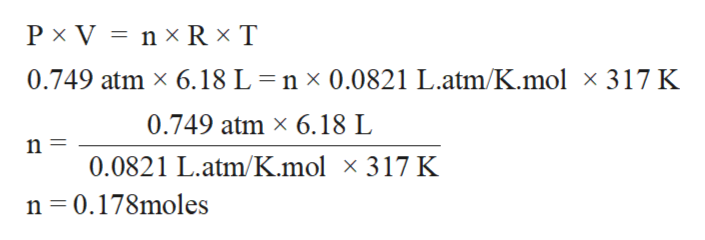# A gas at 569 mmHg and 44.0°C occupies a volume of 6.18 L. Calculate its volume at STP.

Question
1 views

A gas at 569 mmHg and 44.0°C occupies a volume of 6.18 L. Calculate its volume at STP.

check_circle

Step 1

All matter can be classified as solid, liquid and gases mainly. In these physical states of matter, the solid state is composed of particles with least intermolecular distance and strongest intermolecular force of attraction. On the contrary, the gaseous state of matter is composed of particles with largest intermolecular distance and weakest intermolecular force of attraction.

Step 2

The gaseous state is the state of matter with least intermolecular force of attraction between molecules and highest kinetic energy. The standard properties of gases like Pressure, volume, and temperature can be calculated with the help of ideal gas equation.

The ideal gas equation can be written as:help_outlineImage TranscriptioncloseP x V n x R x T Here: P Pressure V volume T temperature R gas constant n moles of gas fullscreen
Step 3

Calculate moles of gas:

Pressure = 569 mmHg  = 0.749  atm ( 1 atm = 760 mmHg...help_outlineImage TranscriptioncloseP x V nx R x T 0.749 atm x 6.18 L = n x 0.0821 L.atm/K.mol x 317 K 0.749 atm x 6.18 L 0.0821 L.atm/K.mol x 317 K n 0.178moles fullscreen

### Want to see the full answer?

See Solution

#### Want to see this answer and more?

Solutions are written by subject experts who are available 24/7. Questions are typically answered within 1 hour.*

See Solution
*Response times may vary by subject and question.
Tagged in

### Ideal gases